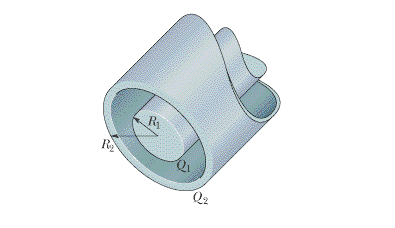# Gauss' Law help

! AHH Gauss' Law!

## Homework Statement

Figure 23-27 is a section of a conducting rod of radius R1 = 1.30 mm and length L = 11.00 m inside a thick-walled coaxial conducting cylindrical shell of radius R2 = 10.0R1 and the (same) length L. The net charge on the rod is Q1 = +3.40 × 10^-12 C; that on the shell is Q2 = −2.00Q1.

a) What is the magnitude and (b) direction (radially inward or outward) of the electric field at a radial distance r=2R2?

What are (c) E and (d) direction at r=5R1?

What is the charge on the (e) interior and (f) exterior surface of the shell?## Homework Equations

I'm going to be honest, I slept through most of this class this week (it's like being in @!#\$ing morgue!) so I am having some trouble here. ANY help is greatly appreciated

I am sure I need some form of Gauss' Law (since that is the name of the chapter).

I am just not sure which form? can someone help me out here?

I know that $\phi=\frac{q_{enc}}{\epsilon_o}$

There are many formulae, but this one seems promising since I know the q_enc.

I also know that by the definition of a flux

$$\epsilon_o\int\vec{E}\cdot d\vec{A}=q_{enc}$$

I am just a little jammed from here. How can I manipulate that integral? Or better still, what can I use instead of the integral so that I can write it as E=...SOMETHING ?

Anybody got a hint?

Marco_84
Anybody got a hint?

think what mean \intE\dotndA... try to find the good coordinates... look the pictutr... and solve your integral...

ciao

Feldoh
Think of the cylinders as lines of charges

erm. outside is conducting so I don't believe it has any electrical field on the inside, please correct me if I'm wrong..but uh, just do the flux = E(2pi*r*l), and then use gauss', treating it as a line of charge (lambda*l)/epsilonknot
do a bit of algebra, treating the center of the inner cylinder as a line of charge with a cylindrical gaussian surface around it and you have E = (lambda/(2*pi*epsilonknot*r))
this should get you started at least.

Think of the cylinders as lines of charges

I know this holds the key to solving this, but for some reason I cannot see it. Man, I wish mt professor gave us some reason to stay awake in class. Do you know how obnoxious it is to listen to a teacher just read straight from the text for an hour and a half! I am sure we all get crappy teachers at some point, but man, this sucks!

hotcommodity
You're asked for the magnitude and direction of the electric field at 2R2, and 5R1, in other words, you want the electric field for a point outside of the outer shell. You can obtain a function for the electric field outside of the outer shell by using Gauss' Law, and appealing to symmetry. Think of a gaussian cylinder that encloses the conducting materials, such that the end caps of the cylinder do not intercept any electric field. In other words, the flux through the endcaps of the gaussian cylinder is zero simply because the $$d\vec{A}$$ pointing outward from each endcap is perpendicular to $$\vec{E}$$ (E dotted with A is zero). Now all you have to worry about is the electric flux through the sidewall of the cylinder. We have:

$$E(r)A_{sidewall} = \frac{Q_{ENCLOSED}}{\epsilon_0}$$

This will be the electric field for a point at a radial distance "r" such that "r" > R2. We already know the enclosed charge, now all we need is the area of the sidewall and we're in business. The area will be $$A = 2 \pi r L$$ . Now all you have to do is solve for E(r) above and plug in the values that you know. Of course, if you find that the electric field yields a negative value, you know it will point inward, and if it yields a positive value, it will point outward.

Feldoh
You're asked for the magnitude and direction of the electric field at 2R2, and 5R1, in other words, you want the electric field for a point outside of the outer shell. You can obtain a function for the electric field outside of the outer shell by using Gauss' Law, and appealing to symmetry. Think of a gaussian cylinder that encloses the conducting materials, such that the end caps of the cylinder do not intercept any electric field. In other words, the flux through the endcaps of the gaussian cylinder is zero simply because the $$d\vec{A}$$ pointing outward from each endcap is perpendicular to $$\vec{E}$$ (E dotted with A is zero). Now all you have to worry about is the electric flux through the sidewall of the cylinder. We have:

$$E(r)A_{sidewall} = \frac{Q_{ENCLOSED}}{\epsilon_0}$$

This will be the electric field for a point at a radial distance "r" such that "r" > R2. We already know the enclosed charge, now all we need is the area of the sidewall and we're in business. The area will be $$A = 2 \pi r L$$ . Now all you have to do is solve for E(r) above and plug in the values that you know. Of course, if you find that the electric field yields a negative value, you know it will point inward, and if it yields a positive value, it will point outward.

Yeah that's essentially about what I was talking^^